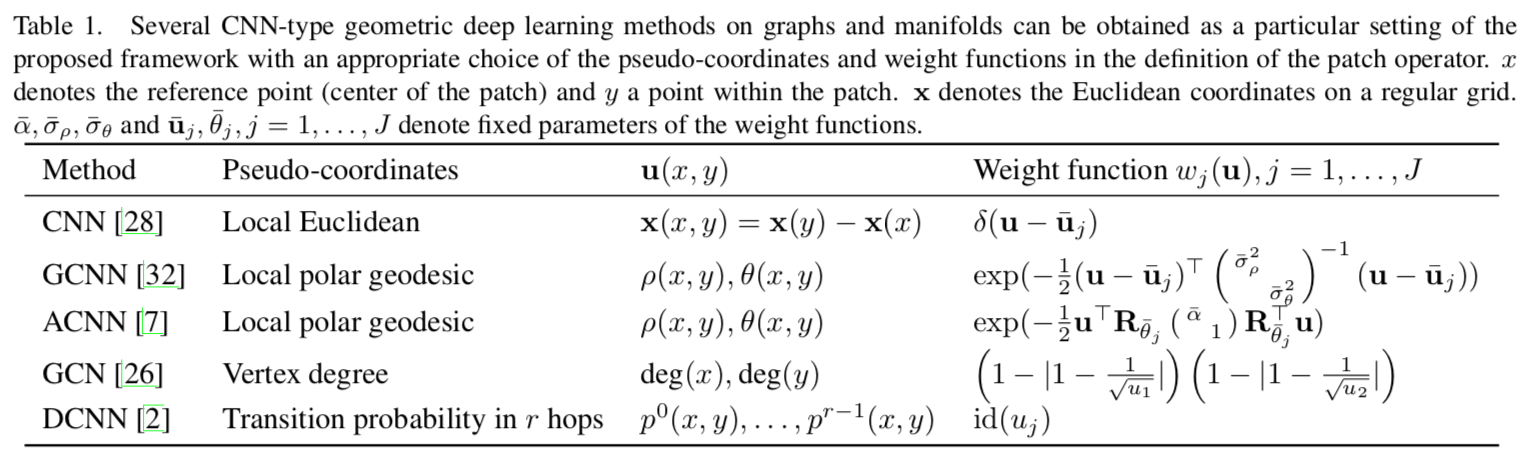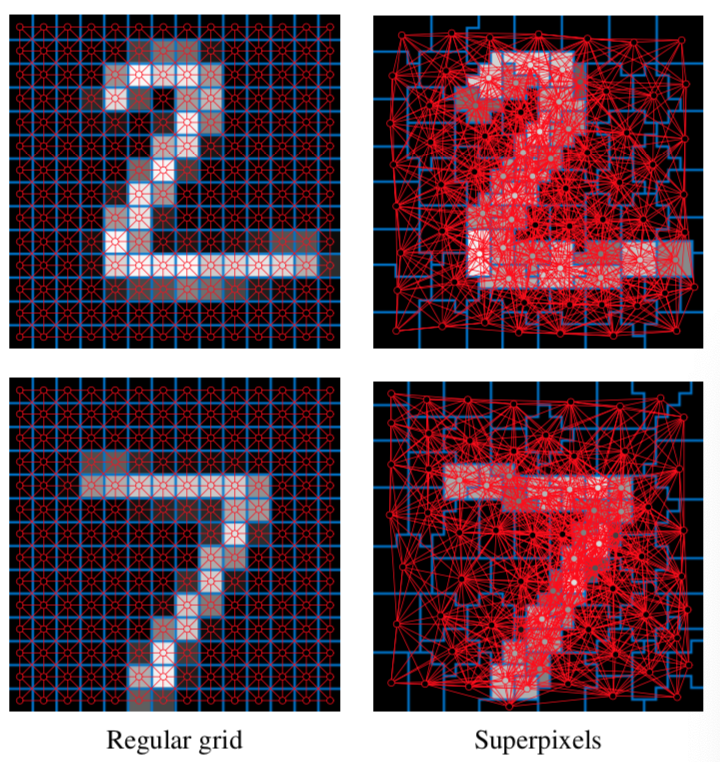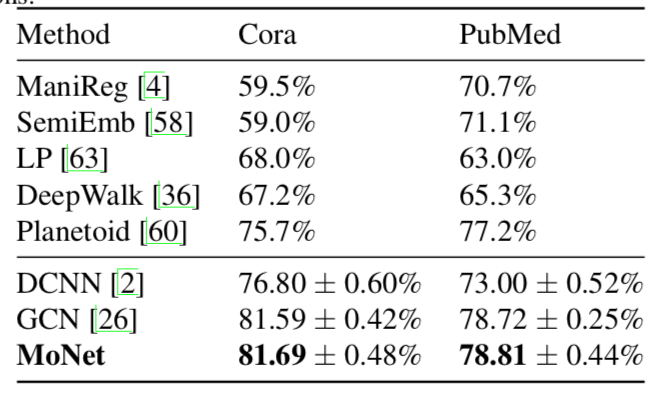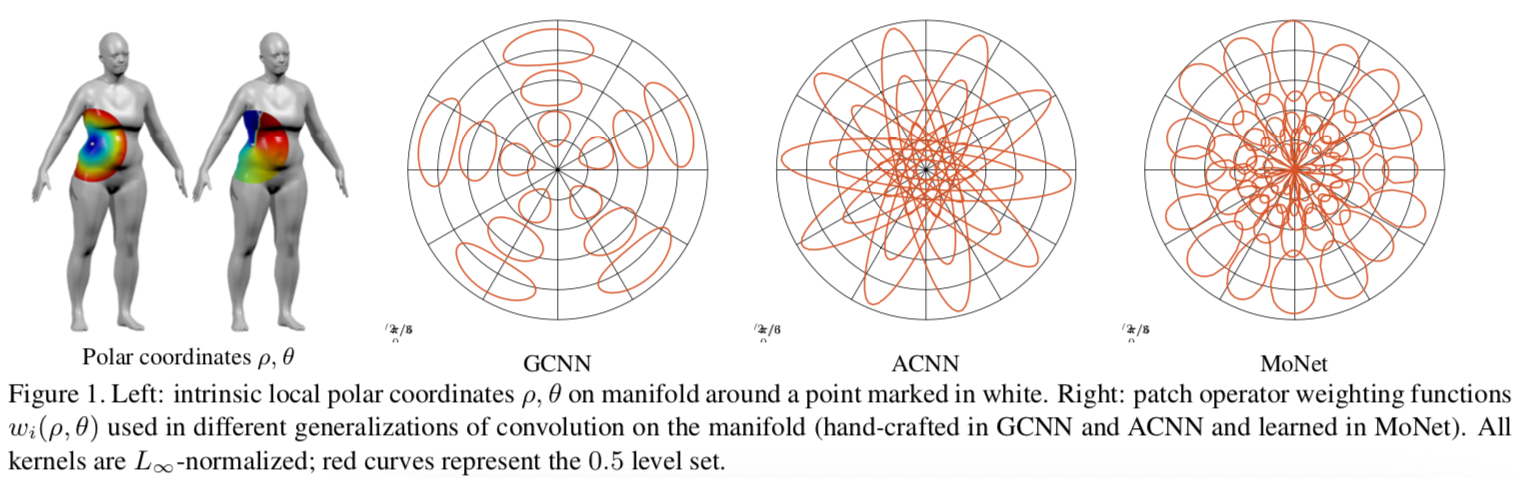# 使用混合模型的卷积神经网络做图与流形的几何深度学习

## 模型

$$D_j(x)f = \sum\limits_{y \in N(x)} w_j(u(x, y))f(y), j=1, \cdots, J$$

$$(f * g)(x) = \sum\limits_{j=1}^{J} g_jD_j(x)f$$

• 其中 u(x, y) 是相对位置/伪坐标，这个设定使得方法适用于流形
• w 将其映射到一个权重上，这样可以在不同位置共享参数u(x, y) in CNN:

(-1, -1) (0, -1) (1, -1)
(-1, 0) x (1, 0)
(-1, 1) (0, 1) (1, 1)

GCN: $$f * g = \theta (I_n + D^{-\frac{1}{2}}AD^{-\frac{1}{2}})f$$

DCNN: $$H^{(k)} = f(W^{(k)} \odot (D^{-1}A)^k X$$

## 实验

### 图片分类LeNet 最好，MoNet 稍差。

### 图链接预测### 流形上的学习